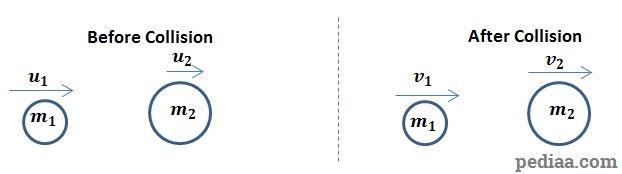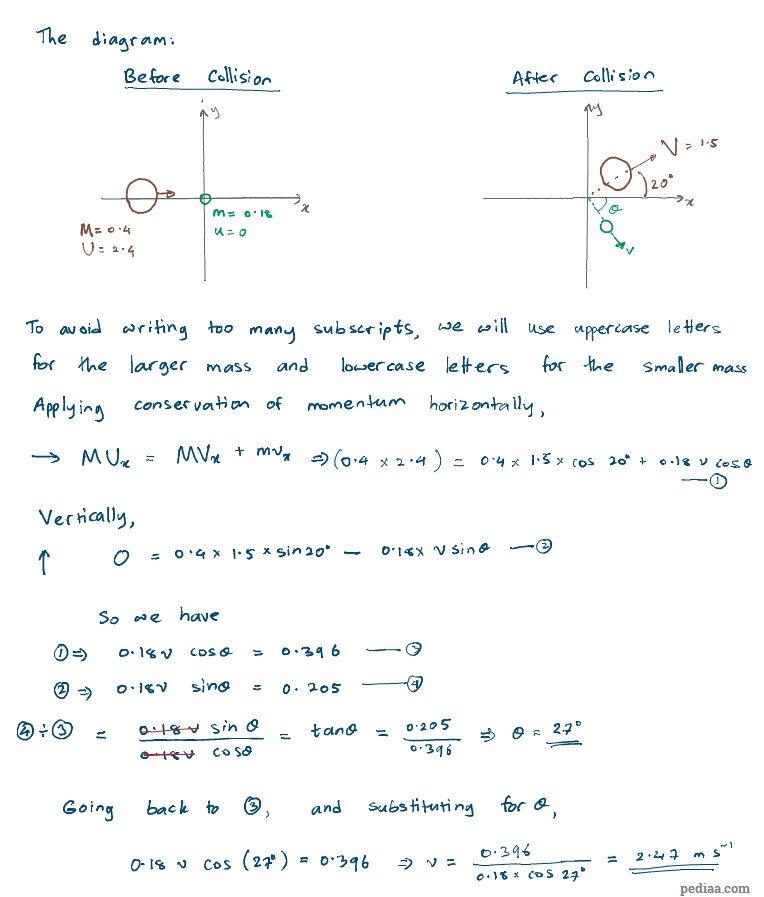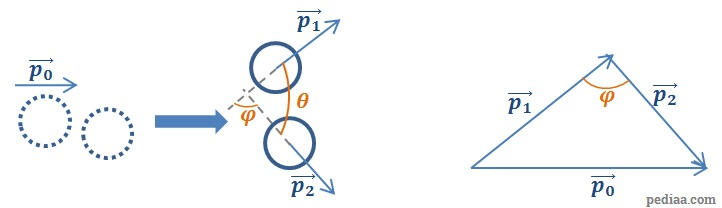# How to Solve Momentum Problems

Here, we will look at how to solve momentum problems in both one and two dimensions using the law of conservation of linear momentum. According to this law, the total momentum of a system of particles remains constant as long as no external forces act on them. Therefore, solving momentum problems involve calculating the total momentum of a system before and after an interaction, and equating the two.

## How to Solve Momentum Problems

### 1D Momentum Problems

Example 1

A ball with a mass of 0.75 kg travelling at a speed of 5.8 m s-1 collides with another ball of mass 0.90 kg, also travelling in the same distance at a speed of 2.5 m s-1. After the collision, the lighter ball travels at a speed of 3.0 m s-1 in the same direction. Find the velocity of the larger ball.How to Solve Momentum Problems – Example 1

According to the law of conservation of momentum,$m_1u_1+m_2u_2=m_1v_1+m_2v_2$.

Taking the direction to the right on this digram to be positive,$\left( 0.75\times 5.8\right)+\left( 0.90\times 2.5\right)=\left( 0.75\times 3.0\right)+\left( 0.90\times v_2\right)$

Then,$6.6=2.25+0.90v_2\Rightarrow v_2=\frac{6.6-2.25}{0.90}=4.8\mathrm{\:m\:s^{-1}}$

Example 2

An object of mass 0.32 kg traveling at a speed of  5 m s-1 collides with a stationary object having a mass of 0.90 kg. After the collision, the two particles stick and travel together. Find at which speed they travel.

According to the law of conservation of momentum,$0.32\times 5=\left( 0.32+0.90\right)\times v$.

Then,$v=\frac{0.32\times 5}{0.32+0.9}=0.31\mathrm{\:m\:s^{-1}}$

Example 3

A bullet having a mass of 0.015 kg is fired off a 2 kg gun. Immediately after firing, the bullet is travelling at a speed of 300 m s-1. Find the recoil speed of the gun, assuming the gun was stationary before firing the bullet.

Let the recoil speed of the gun be$v$. We will assume the bullet travels in the “positive” direction. The total momentum before firing the bullet is 0. Then,$0=\left( 0.015\times 300\right)+\left( 2v\right)\Rightarrow v=-\frac{0.015\times 300}{2}=-2.25\mathrm{\:m\:s^{-1}}$.

We took the bullet’s direction to be positive. So, the negative sign indicates that the gun is travelling in the answer indicates that the gun is travelling in the opposite direction.

Example 4: The ballistic Pendulum

The speed of a bullet from a gun can be found by firing a bullet at a suspended wooden block. The height ($h$) that the block rises by can be measured. If the mass of the bullet ($m$) and the mass of the wooden block ($M$) are known, find an expression to calculate the speed$u$ of the bullet.

From conservation of momentum, we have:$mu=\left( m+M\right)v$ (where$v$ is the speed of the bullet+block immediately after collision)

From conservation of energy, we have:$\frac{1}{2}\left( m+M\right)v^2=\left( m+M\right)gh\Rightarrow v=\sqrt{2gh}$.

Substituting this expression for$v$in the first equation, we have$mu=\left( m+M\right)\sqrt{2gh}$$\therefore u=\frac{\left( m+M\right)}{m}\sqrt{2gh}$

### 2D Momentum Problems

As mentioned in the article on the law of conservation of linear momentum, to solve momentum problems in 2 dimensions, one needs to consider momenta in$x-$ and$y-$ directions. Momentum will be conserved along each direction separately.

Example 5

A ball of mass 0.40 kg, traveling at a speed of  2.40 m s-1 along the$x-$ axis collides with another ball of mass 0.22 kg traveling at a speed of  mass 0.18, which is at rest. After the collision, the heavier ball travels with a speed of 1.50 m s-1 with an angle 20o to the$x-$ axis, as shown below. Calculate the speed and direction of the other ball.How to Solve Momentum Problems – Example 5

Example 6

Show that for an oblique collision (a “glancing blow”) when a body collides elastically with another body having the same mass at rest, the two bodies would move off at an angle of 90o between them.

Suppose the initial momentum of the moving body is$\vec{p_0}$. Take the momenta of the two bodies after the collision to be$\vec{p_1}$ and$\vec{p_2}$. Since the momentum is conserved, we can draw up a vector triangle:How to Solve Momentum Problems – Example 6

since$\vec{p}=m\vec{v}$, we can represent the same vector triangle with vectors$m\vec{v_0}$,$m\vec{v_1}$ and$m\vec{v_2}$. Since$m$ is a common factor to each side of the triangle, we can produce a similar triangle with just the velocities:How to Solve Momentum Problems – Example 6 Velocity vector Triangle

We know the collision is elastic. Then,$\frac{1}{2}m{v_0}^2=\frac{1}{2}m{v_1}^2+\frac{1}{2}m{v_2}^2$.

Canceling out the common factors, we get:${v_0}^2={v_1}^2+{v_2}^2$

According to Pythagors’ theorem, then,$\varphi=90^o$. Since$\varphi +\theta=180^o$, so then$\theta=90^o$. The angle between the two bodies’ velocities is indeed 90o. This type of collision is common when playing billiards.